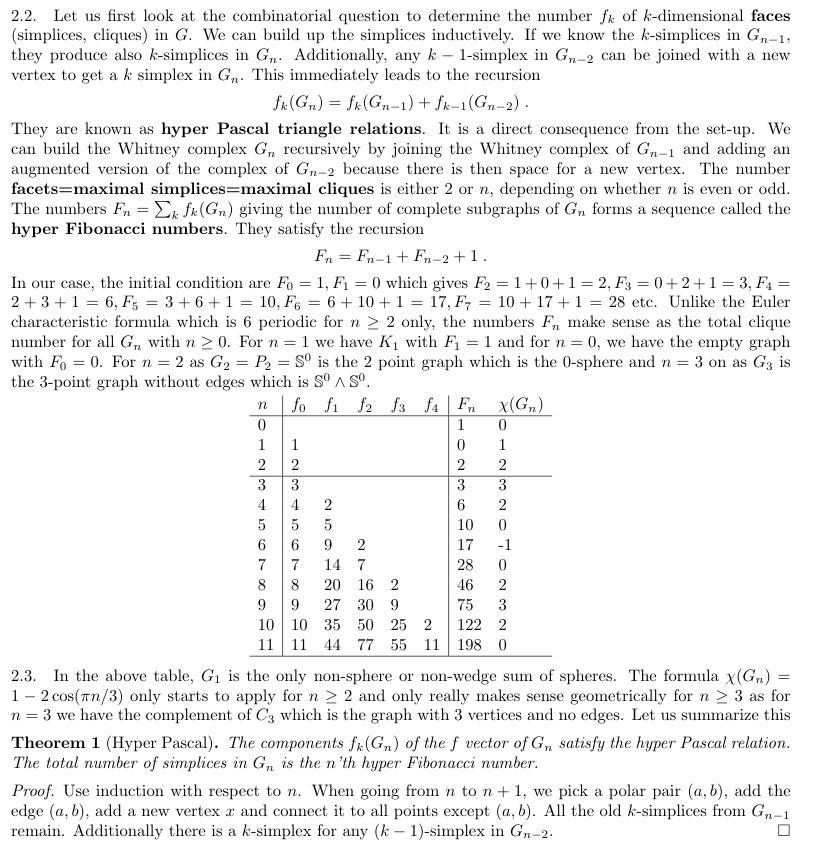# Math E-320 Blog

Previous blogs: 2017, 2016, 2015 (fall), 2015 (spring), 2014, 2013, 2012, 2011, 2010.

#### Lecture 13: Computer science

Here is a pre-lecture showcasing 10 spots in computer science
We focused on 10 topics in computer science:
Topic Example
Experimental mathematics Gaussian Primes or Experiments in number theory
Artificial intelligence Sofia project fall 2003, spring 2004, or Math Circle Talk Notes, 2007
Algorithms Simplex generating function
Limits of computing A turing machine animation (2000)
Complexity theory Scene from Travelling Salesman movie
Languages Data Structures Examples of programming languages
Inverse problems An example of an inverse problem
Computer vision Omni Direction vision and Structure of motion
Network structures Graph geometry project
Computer museum TI 59 calculator hack

#### Lecture 12: Dynamical systems

In the second last lecture, we look at dynamical systems. The theory of dynamical systems is the theory of ``time" and ``time evolutions". As illustrated during lecture there is a huge fascination with the concept of ``time":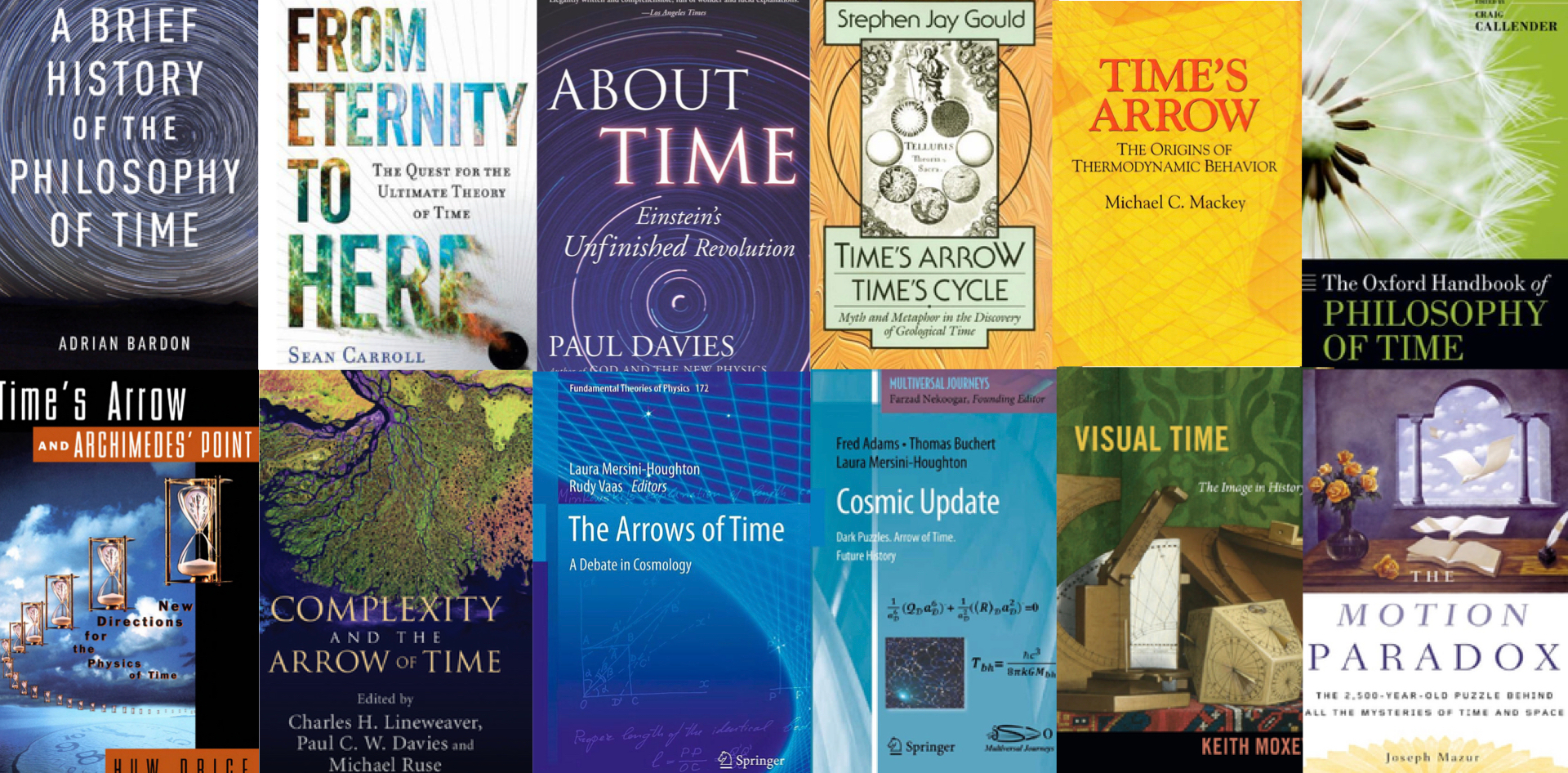For a mathematician, time is just an operation of natural numbers or positive real axes on a space. For every integer or real number we get the position x(t) at time t. We either have a discrete rule which maps a state x to a new state T(x) or then we have a differential equation which produces a continuous deformation x(t) of the state. In the first case, time consists of the natural numbers, in the second case, time is the positive real axes. Sometimes, dynamical systems also can be reversed but most of the time we can not. The motion of the planets or the Schroedinger evolution of a quantum state are examples which can be reversed. Other systems like heat evolution, diffusion or many number theoretical evolutions like the Collatz map T(x)=x/2 if x is even and T(x)=3x+1 if x is odd are not reversible.
Especially intriguing when looking at time evolutions is that even so everything is completely deterministic, the system can produce chaos: the system can be proven to generate randomness. A prototype is T(x) = 4x(1-x), which is a map on the interval [0,1]. If you apply this map T for 60 times on a starting number like x=0.4, then the outcome is different than if you use the map S(x) = 4x-4x2 with the same starting number. While T(x)=S(x), obviously, it is no more true that T60(x) = S^60(x) if you compute this on a computer. You can try this out with this Desmos applet I have written for a data project in this single variable course. Go to that applet, you see that T60(0.81) = 0.93295901419. If you change the function to S(x) = 4x-4x2, then S60(0.81) = 0.451831018858. This is not a problem with Desmos. Any programming language working with say 16 digit accuracy has this problem. The reason is that the map T is chaotic. Its Kolmogorov Sinai entropy is Log(2) meaning that in average, errors double in each step. Now, 260/1017 is larger than 1 meaning that a small initial change (and a swap from T(x)=4x(1-x) to S(x) = 4x-4x2 produces such variations on the level of the accuracy) produces an error which is macroscopic already after 60 steps. If we would use 100 digit accuracy for the computation, we would would see the same after 330 iterations.
In the pre-lecture, we look at 10 dynamical systems and open problems. Some things appear already in a talk on "intriguing open problems in Hamiltonian dynamics from October 19, 2000 given in the beautiful town of Bozeman in Montana. Then, 20 years ago, I had used Mathematica 4 and Povray 3.0. Now, things were done in Mathematica 12 and Povray 3.7. I made use of some work done for the website dynamical-systems.org which I wrote in 1999. For Vlasov, see this existence result or Section 5.4 in this book.
 System Time Space Unsolved Simulation Chirikov Z 2-torus Entropy ≥ log(c)/2? Javascript (2015) n-body problem R R6n Global existence a.e. Javascript (2000) Billiard Z Cylinder Positive entropy possible in smooth case? Some pictures Double pendulum R M in R4 Positive entropy? Javascript Double Pendulum Outer billiard Z plane Stability for C1 curves? Exterior billiard Cell. automata Z AZd Transitivity implies Devaney chaos? CA Javascript 2015 Vlasov system R smooth diffeos: R2d macrosopic part settles to equilibrium Vlasov Javscript 2000 Rigid body R TSO(3) positive entropy case? Which integrable Rigid body povray Logistic map N [0,1] dimension of Feigenbaum attractor Graphics (PNG) Collatz N N {1} is the only attractor Graphics (PNG)
A panorama from the science center (click for a large 11K pixel panorama)P.S. on a bit more meta level: I had been asked what the purpose of the pre-videos is. One of the things I consider extremely important in teaching is that one gets into a class not only prepared but also pepped up and energized, especially if one has taught something a lot already. This means starting to think about a subject several days before. Doing these pre-lectures is for me also an opportunity to push the envelope in using technology for teaching. This year, I explore especially the use of demonstration objects in video. This has been done before for expensive TV productions. New is that it is possible today to do this alone with relatively little short time (in this case maybe 40 hours of work for a 15 minutes of video, with a 18 hour essentially non-stop finish done on Saturday for recording and cutting). This is not counting the hundreds of hours of programming which in this case, as I reuse and reran with better quality also code I have written over decades. This was done over a couple of days. Some of the animations take hours to complete. The final movie (15 minutes) was 59 Gig big when submitted to youtube. The final cut directory has 357 Gig (1/3 of a TByte). The source videos (which are kept separate are an additional 140 Gig. In total, this small project alone covers 550 gig in my macmini (the cheapest version with 256 Gig harddrive which I had to buy in an emergency, due to a machine failure). Of course, this requires to work on external drives all the time. Since also the source files are large, the entire process needs to be orchestrated, working on different things at the same time, like when waiting to render the 360 degree camera stuff or then for copying the files. And then things need to be backed up as even modern stuff can have hick ups. Like in rock climbing, where at any time two body parts have to be on the rock, I keep at any moment of time always two copies. There is an exception just when recording but that is, where the multiple cameras come in. Even in the case of an SD card or camera failure, the other camera footage could still work. I don't have time for a second shot and a fixed time window in which this has to be done (we get access to the department by preregistration and of course by testing). Why not at home? Well that needs negotiations with the partner.
Here is a Video of a coupled systems of oscillators, found by Stephen.

#### Lecture 11: Cryptology

Cryptology is a part of mathematics which affects us daily. We will look at various encryption systems. We use pretty much a chronological approach starting with simple alphabet swaps and reach modern systems like RSA or DES.
Cryptology appears at first more to be something like an engineering task. Indeed, crypto systems like DES are rather arbitrary. However, more modern crypto systems which are based on modular arithmetic are more natural, more beautiful, more mathematical and related to fundamental questions in mathematics: how fast can we factor integers in principle? We currently do not know how to factor say a 1000 digit integer that is the product of two 500 digit primes. An other unrelated question is how fast we can solve the logarithm problem in a finite field: if ax = b mod (p). How do we compute x = loga(b)? Also this problem appears to be difficult and can not be tackled say for a 1000 digit prime p. Both of these problems are fundamental obstacles of cracking RSA. The discrete log problem also appears in the Diffie-Hellman key exchange system. Both of these fundamental problems are natural. An other society on an other planet would probably come up with these systems too. They would however very unlikely invent something as ugly as the data encryption standard DES. There would be much more to cryptology. The subject has moved much also to algebraic geometry with the advent of elliptic curves as cryptosystems. The idea is the same. Rather than using the multiplicative group of a finite field, one can use elliptic curves over finite fields and do exactly the same. One can use elliptic curves also to factor integers.
In the lecture, we look at 10 crypto systems
 Crypto system Discovery Math Polybius 300 BC Alphabet swap Scytale 700 BC Scrambling of entire text Caesar 70 BC Alphabet permutation Trithemius 1508 Polyalphabetic substitutions Viginere 1553 Polyalphabetic with key Ottendorf 1780 Book cypher (common reference) Enigma 1918 Polyalphabetic with dynamic swap RSA 1977 Modular arithmetic, Factoring Diffie-Hellman 1976 Modular arithmetic, discrete log DES 1970 Block cipher
Some comments on the video. The drone footage was also filmed on Saturday. The drone flew to the quad losing there connection (it then starts to return by itself). The app complained about radar and other interferences. Definitely, in urban areas, the flight distance can be dramatically reduced. The 5 mile distances seen on youtube videos happen in relatively deserted areas.

[Something unrelated and a bit funny: youtube itself is a crypto riddle. The ``like counter" does currently not work on this one. I know that because some family members who are among the handful of my ``fans" are not able to cast their vote on this movie. Such an incidence doesn't boos confidence in social media metrics. Of course, for me as a teacher this does not matter as I just need to reach my students, but for a business, metrics can be important. How do we know social metrics work? In my case, with single digit likes, I can experimentally check whether it does, in general, we have to trust. Here is a screen shot of the stuck counter.]

#### Lecture 10: Analysis

Analysis is a huge area of mathematics. It contains subfields like real and complex analysis which is calculus using real or complex numbers, then Harmonic analysis which deals with decompositions of functions into natural components, then partial differential equations which looks at time evolutions of spacial extended objects, then functional analysis which deals with spaces of functions containing typically very strange ones, like the Weierstrass curve as an example of a continuous function. Then there is the calculus of variations which looks at objects minimizing a quantity. Extremization is a principle on which one can build essentially all laws we know describing nature. Sometimes these extrema are nice like sphere minimizing the enclosed surface area for a fixed surface area. With some constraints the minima can already become more interesting are in general not smooth any more and there are many extrema which are of fractal nature, like the path traced by a lightening flash. In the movie recorded on Easter in my office, we cover 10 fractals:
 Fractal Discovery Dimension Math 1) Cantor-Smith Set 1874-1883 log(2)/log(3) 2-adic integers 2) Koch snowflake 1904 log(4)/log(3) dimension theory 3) Sierpinski carpet 1916 log(8)/log(3) universal for 2d curves 4) Menger sponge 1926 log(20)/log(3) universal for 3d curves 5) Weierstrass function 1872 1-log(a)/log(b) harmonic analysis 6) Lorenz attractor 1963 numerical 2.4 differential equations 7) Diffusion limited aggregation 1981 numerical 1.71 stochastic processes 8) Barnsley fern 1988 1.45 L-systesms, IFS 9) Mandelbrot set 1978-1980 2 Complex dynamics 10) Mandelbulb 1988-2009 ? Spherical geometry
There would have been many more to mention. Good to include would have been the Hofstadter butterfly, the burning ship, the mandelbar, some Kleinian group fractal, some limiting perculation clusters, some phase space fractal chaotic sea in Hamiltonian dynamics or the KAM attractor or then say more about Brownian motion or cantor spectrum for operators. Well, maybe something for an other time.
In the description of the movie, we mention that Povray allows to compute the Mandelbrot set in 2 lines. Here is an example:
```camera{orthographic location <-1,0,-3>/2 look_at <-1,0,0>/2}
plane{z,0 pigment{mandel 100 color_map{[0 rgb 0][1/6 rgb <1,0,1>][1/2 rgb 1][1 rgb 0]}}}
```
It is a solution to the homework assignment to produce a picture of the Mandelbrot set. I personally am a big fan of short code. A programming language needs to be designed in such a way that one can do things with little amount of code and as little libraries. If possible no libraries at all. I program since 45 years. Libraries are the main reason why programs start to fail, especially after some time. An other poison is redesign of the language making standard things obsolete. Worse even are small changes of routines. I love the programming language C, but already there, the use of libraries can be a curse. Look at Hello World in C:
```#include
int main() { printf("Hello, World!"); return 0; }
```
In comparison, the above two lines of Povray code produce a movie of the Mandelbrot set in which the level surfaces and color are adapted to the zoom level. It places the mandelbrot set onto a plane and then films it moving the camera closer and closer. Here is a 3 line code for Mathematica. It does not produce a movie yet, but just a single frame:
```F[c_]:=Module[{z=c},Do[z=N[z^2+c];If[Abs[z]>3,z=10],{100}];If[Abs[z]>3,0,1]];
M=500; N[9*Sum[F[-2.5+3 Random[]+I(-1.5+3 Random[])],{M^2}]/M^2]
S=M=300;A=Table[F[-2.5+3k/M+I(-1.5+3l/M)],{l,M},{k,M}]; ListDensityPlot[A,Frame->False,Axes->False]
```

#### Lecture 9: Topology

In the topology lecture, we look at `rubber geometry". Traditionally we have in this course focused class work on polyhedra and Euler characteristic. This is not completely off. The birth crib of topology are graphs (Koenigsberg bridge problem) and algebraic topology essentially makes simplicial decomposition to a tool allowing to prove things. Discrete approximations of the continuum are surprisingly accessible, illustrated by the fact that Platonic solids were considered very early in mathematics. So, rather than look at the 10 open problems like in number theory or 10 miracles like in geometry or 10 results in set theory or 10 stories in probability theory, we look at the 10 most beautiful objects in topology. Topology is closely related to visual art.
 1 sphere (hopf fibration as a visualizatiion of the 3-sphere) 2 torus (3d Torus visualization as the office with opposite sides identified) 3 polytopes (all 6 regular platonic 3-polytopes, 5,8,16,24,120 and 160 cells) 4 varieties (we see a Decic and a Calabi-Yau) 5 moebius strip (build it with clay) 6 klein bottle (we build it with pledo) 7 jordan curve (peano type case) 8 antoine's neckless (and alexander sphere) 9 knots (no knots in 4 dimensional space) 10 networks (graphs, we see 100 fullerene)
Here is a screen shot of a recording done in my office and the board photo (click for larger versions):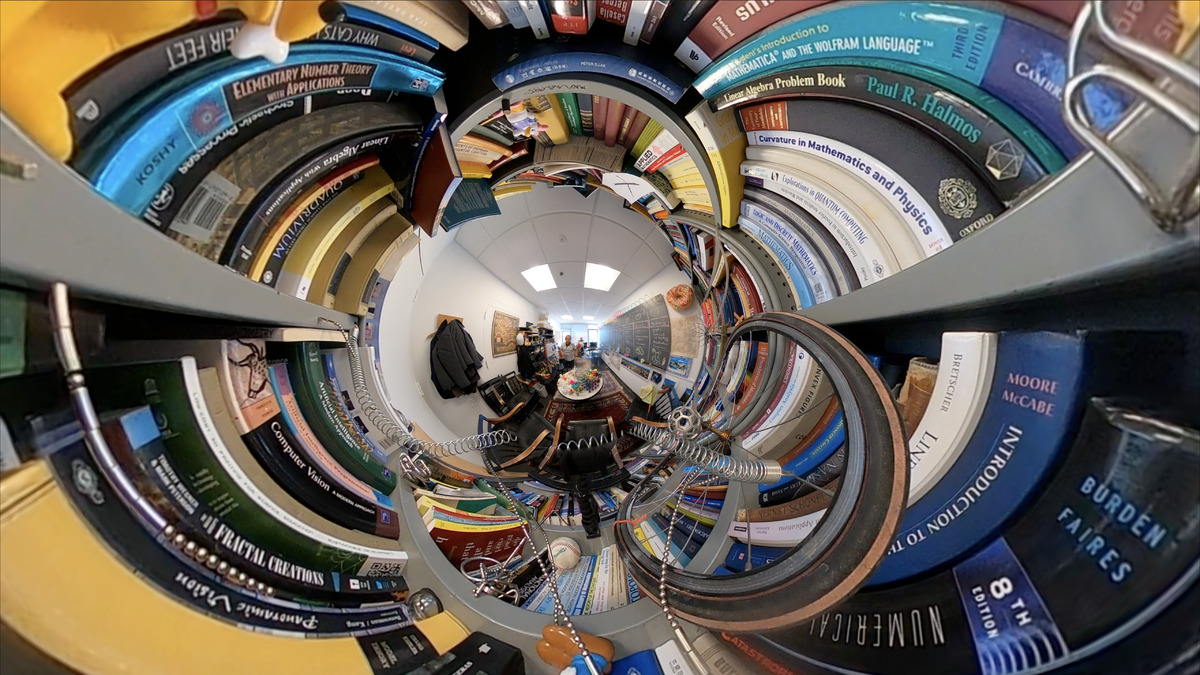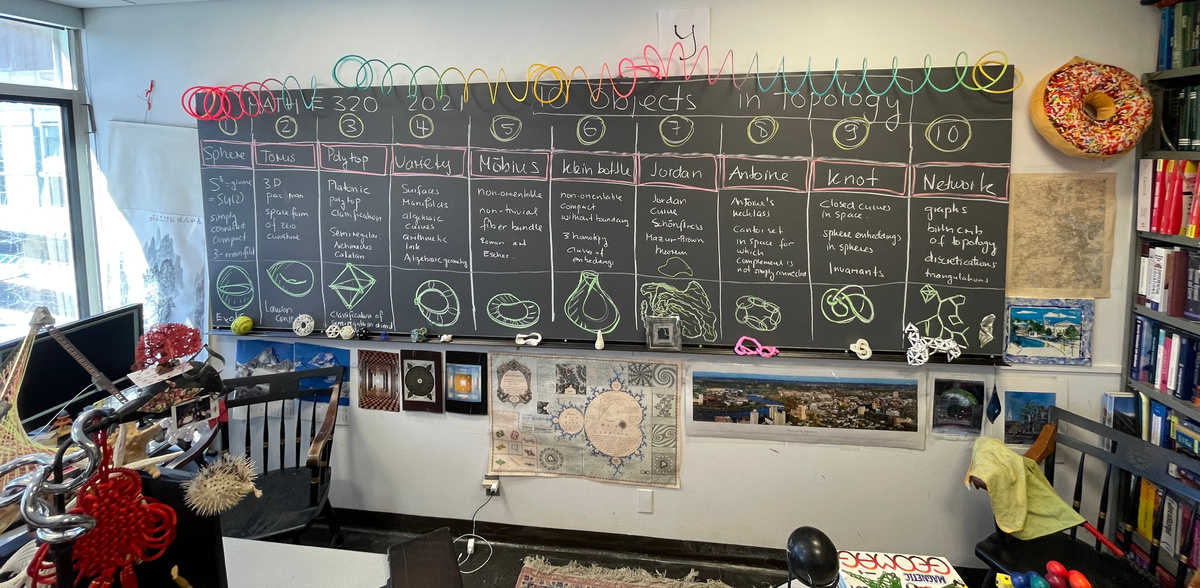#### Lecture 8: Probability theory

We look at probability theory. In number theory, the open problems were most exciting, in set theory the theorems. In probability theory it is the huge amount of popular stories which make it exciting. We tell 10 stories in 22 minutes: Update May 16, 2021: Numberphile has a longer 20 minute video on the doubling strategy. One always points out that the odds for red and black are not equal. But that does not skew the argument: You win with the Martingale strategy (in a mathematical sense) also if the odds are 10 to 1 or a million to 1 against you! You just always double until you win. The funny thing is that the topic is more psychological: the martingale problem is obviously a mathematical problem but people always refer to the practical implementations (the bank has a finite amount of money or betting limits). That is not the point. It is like you would argue the harmonic series converges because it is not possible to get in any reasonable time to 1000 by counting up (which is also a practical implementation. The silly fact is that there, the practical limitations are never pointed out. The mathematical fact is that if the doubling strategy works and the math is very simple as the Numberphile video explains well. There is an other interesting, more psychological point. I used in my video 100 dollars and not 1 pound like in the Numberphile video. But if you bet with 1000 dollars then you win with a reasonable chance 1000 dollars within a few games. The chance of dying by some disease or having an accident is bigger. Now, on a grand scale, the martingale strategy is used in finance all the time and if you gamble with a lot of money, you also win big (usually!). There of course, the odds of losing are almost zero if you are attached to a hedge fund with extremely fast efficient trading possibilities.
 1. Martingale Strategy A fool proof winning strategy using the doubling strategy. 2. Conditional probability Dave has 2 kids, one is a boy born at night. Chance that 2. is a girl? 3. Stochastic processes We demonstrate Polya's return theorem using a drunken drone. 4. Integral geometry Throwing the Buffon Needle. Tip of an iceberg for Monte Carlo computation. 5. Decision theory Petersburg paradox. We would not pay 10, but the win expectation is infinite. 6. Axiomatic set-up Kolmogorov did here what Euclid did for geometry. We see the Bertrand paradox. 7. Coincidences, Collisions Birthday paradox For 23 kids, the probability that 2 have same birthday wins. 8. Pseudo randomness Is pi random? Throwing dice, drawing Bingo balls. Pollard 1975 method. 9. Entropy principle Important distributions maximize entropy. Galton board illustration. 10. Quantum mechanics Built into the theory. God does not play dice? The Schroedinger bear.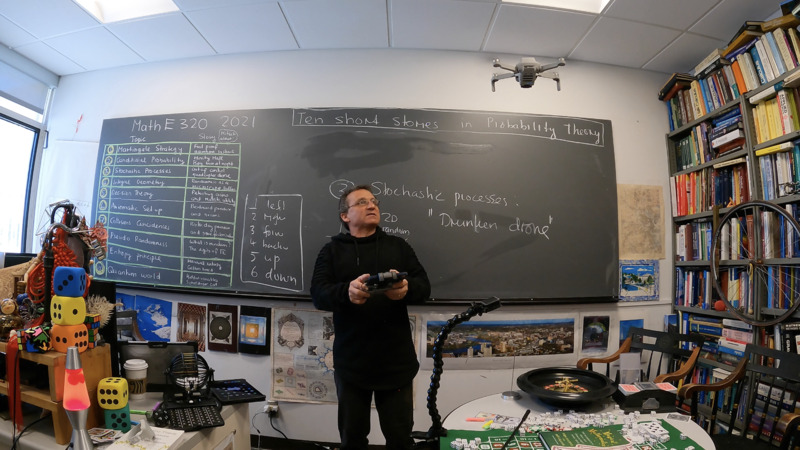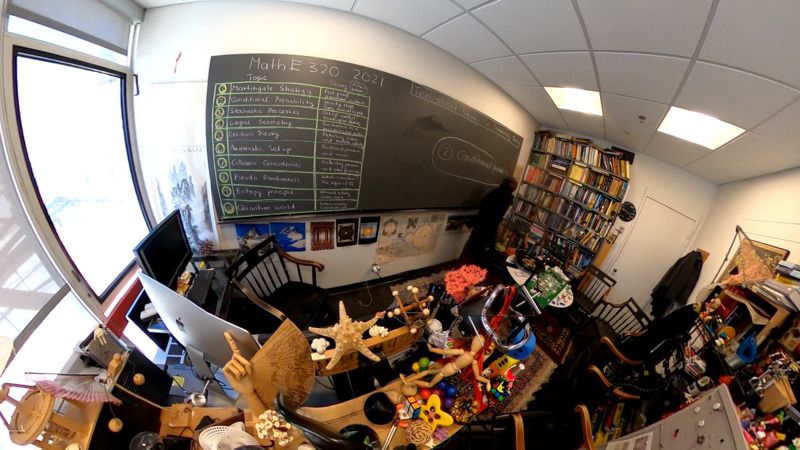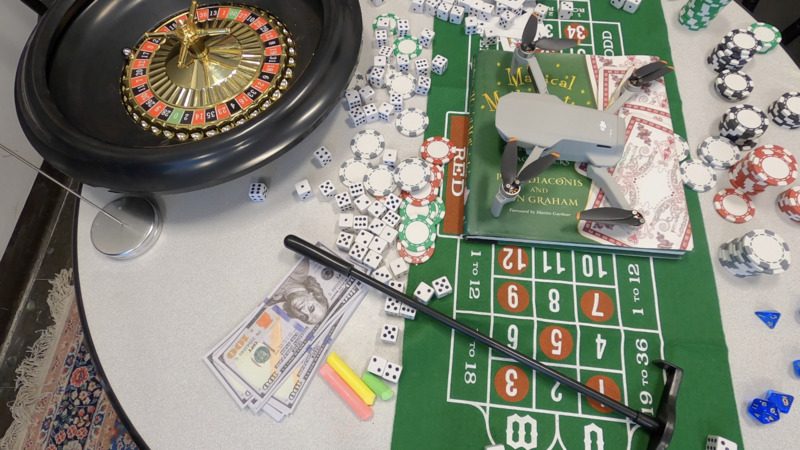Each of the stories might be a bit worn out by itself. But as a whole, the collection however illustrates the richness of the theory. Let me mention something related: humans assess risks or probabilities rather poorly in general. The concept of risk is at the heart of probability theory, statistics and interpretation of data. Game theoretically it is the question how much we want to pay for an expected item. In real life, we dismiss seemingly small risks which could be devastating (like nuclear war, asteroid hit, climate change) and overestimate temporary risks (terrorism 911), communism ( McCarthy), fear of foreign cultures (racism), nuclear war in 1950ies (duck and cover) or a rush into wars (usually enthusiastically supported by the population and also by news media). Probability theory allows to cut through this by building models based on data gathered from history and experience. For example, since nuclear weapons were put in place, there were several close calls. In cases like Able Archer or Cuba, there was one person standing between a nuclear catastrophe. We completely ignore such risks these days. (Maybe not: there is just a story about this) On the other hand, we tend to hype or over-estimate other dangers. Switzerland is still in a lock-down mode, despite the fact that the mortality rate has dropped far below average also for 65+ year old people and the 0-64 year olds wever never, ever in any danger during the entire last year. Switzerland has probably the world best statistical data (and fantastic statisticians, many educated at stellar universities like ETH). Lets look at the excess death data there:In the US, our underfunded CDC does not release such data. Mortality data like climate data are very reliable and look similar in similar countries. The cause of death is a matter of interpretation which is illustrated by the vast fluctuations between different countries. Excess death data correlate well with real dangers and current state of health care systems. Here are the data from the Swiss statistics bureau. But as the above stories in probability theory show, risk and decision making is not the strongest ability of humans. Collectively we are dumb when it comes to making good choices. Policies are done in a social context (peer pressure and fear), game theoretical phenomena (strong, hawkish, repressive and tough leaders tend to be popular and push each other into a Nash equilibrium of doom), censorship effectively enforced today by social media as well as in academia. To the later, I personally got reported by an anonymous coward for commenting on public data last year. Crisis always brings out the worst of humanity), self-interest (news outlets, rich people (me included as I have a job, a roof above my head and enough cash to buy books and electronic equipment to teach), or big technology companies are all winners of the current crisis). But the situation has deteriorated for others. We have a socially accepted path to even bigger income inequalies. Issues like failing education, devastated existences and job losses, women rights, domestic violence surge, fatalities due to untreated other conditions, depression (tripled) due to isolation and drug use surge, crumbling infrastructure (like failing power grids) and lack of money for health care (funds for modernizing health care are even less likely allocated in the future). bankruptcies, tumbling economies worldwide, leading to migration crisis, or ignoring climate issues and avoiding public transport systems, genocides, or wars are ignored. We are so dumb when it comes to assess risks. No, I myself am not smarter. I would probably join similar decisions than current leaders, simply because there is no choice in a Nash equilibrium, where dissent leads to removal and scientific discussion is shouted down (I'm ashamed to call me a scientist these days where data are ignored, and news outlets shamelessly use phrases like ``experts say" without reference nor data backup. All principles we indoctrinate students about data (where do they come from, how were they obtained, how reliable are they, what is cause and what is effect are completely ignored). It is a devastating multiple prisoner dilemma, as modeled in game theory or evolutionary dynamics. Scientists will analyze the current situation more calmly in the future and hopefully find a way to avoid the same mistakes. But enough of this: also the 20th of march was a great day. First day of spring coming up. A panorama from the science center (click for a large 11K pixel panorama)#### Lecture 7: Set theory

During class, when discussing paradoxa, Stephen pointed out the star trek episode with the liars paradox Just added it to the math in movies collection. As always, also this set theory lecture" is split up into sections. We discuss the algebra of sets, infinities, paradoxa and incompleteness. The topic is very much at the heart of the foundations of mathematics. There is not only philosophical drama (are our foundations solid?) but pedagogical dispute (like "new math") or more recently the infiltration of category theory into mathematics and the question whether it can replace set theory as a foundation. There are immediate gems to be harvested from set theory: like that there are different types of infinities. Here is my personal top 10 list (as of now):
 Rank Result Pop Culture 1. Incompleteness theorem Goedel-Escher-Bach 2. Uncountable reals Aleph n bottles of beer on the wall! 3. Cantor-Schroeder-Bernstein Infinity plus one is infinity 4. Well ordering theorem Greatest Math Controversy of all times 5. Boolean Algebra Everything is zero's or ones 6. Laws of thought Thieves are humans, ergo, great thieves are great humans 7. Sets are universal Venn Diagrams: New math! 8. Russel's paradox "I always lie!" 9. Cantor pairing Hilbert's Hotel 10. Independence of C and CH Banach-Tarski
click for larger (4K or 11K) pictures.Like many insights and examples, the example ``thieves are humans, ergo, good thieves are good humans" is from Ernst Specker, from whom I learned linear algebra (2 semesters), logic and model theory (and much more as his lectures were unscripted, and full of little gems). Teachers at the Poly like him made studying math valuable. Content can be read or acquired everywhere; taste, humor and insight and guidance what to look at and what not, needs good teachers.
The 13th was again a wonderful day. Just one day before Pi day. A panorama from the 5th floor science center (click for a large 11K pixel panorama)#### Lecture 6: Calculus

As mentioned in the preparation lecture recorded in my office, there are many of heavy books on calculus. How can we discuss such a huge subject in a 2 hour class? History also here softens the blow. There are many interesting stories starting with the Greeks (Zeno, Archimedes). The fact that much of calculus is very accessible is the huge impact it makes on pop culture which by definition makes it accessible for a larger audience:
 Rank Result Pop Culture 1. Fundamental theorem of calculus Newton-Leibniz rivalry, Newton apple, gravity 2. Euler formula ei π+1=0 most beautiful formula in math 3. Intermediate value theorem Wobbly table, catastrophes, chaos 4. Euler formula Basel problem ζ(2)=π2/6, Riemann hypoth. or ζ(-1)=1+2+3+4+...=-1/12 5. Volume of sphere Archimedes: cone complement in cylinder, Heureka! 6. Harmonic series ζ(-1)=product 1/(1+p) over primes, stacking books 7. Irrational π movie Pi, open problem: is πe rational? 8. Golden key, Partition function primes, man who knew infinity. 9. Fourier series MP3, JPG, MPG image and movie compression 10. Weierstrass function Fractals, most things are not smooth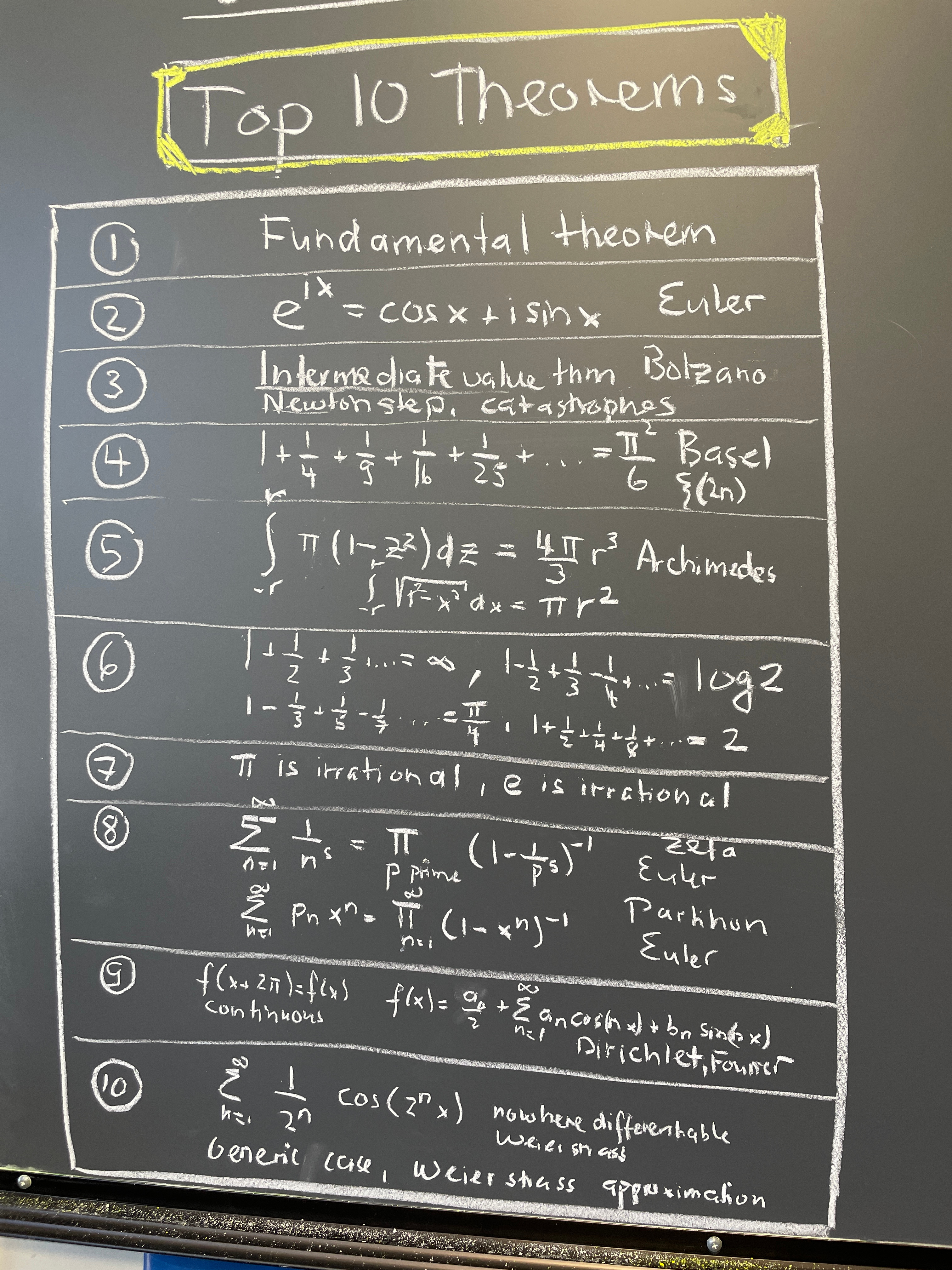click for larger picture.
It was a beautiful day when recording the lecture. Here is a view from the 4th floor science center (click for a large 11K pixel panorama)#### Lecture 5: Algebra

 In the algebra lecture, we will make a bridge from solutions to quadratic, cubic, quartic equations to group theory and puzzles like the famous Rubik cube. While the solutions of the quadratic equation x2+bx+c=0 is well known as 2x=-b+(b2-4c)1/2 and 2x=-b-(b2-4c)1/2, how was the more complicated formula for the cubic equation x3 +ax2+bx+c=0 obtained? How did Cardano get to the seemingly insane solution formula: ```6x=-2a+(2*2^(1/3)*(a^2-3b))/(-2a^3+9ab-27c+3Sqrt*Sqrt[-(a^2 b^2)+4b^3+4a^3c-18abc+27c^2])^(1/3)+ 2^(2/3)* (-2a^3+9ab-27c+3Sqrt*Sqrt[-(a^2 b^2)+4b^3+4a^3c-18abc+27c^2])^(1/3) ``` What are the corresponding formulas for the quartic or quintic? This brings in symmetries and group theory. We will look at a zoo of Rubik type puzzles. Here is a computation of its size of the classical Rubik cube with GAP. This puzzle could be related to a polynomial of degree 48 (there are 6 faces, each having 8 colored stickers and these places are permuted when playing the game). ```rubik := Group( ( 1, 3, 8, 6)( 2, 5, 7, 4)( 9,33,25,17)(10,34,26,18)(11,35,27,19), ( 9,11,16,14)(10,13,15,12)( 1,17,41,40)( 4,20,44,37)( 6,22,46,35), (17,19,24,22)(18,21,23,20)( 6,25,43,16)( 7,28,42,13)( 8,30,41,11), (25,27,32,30)(26,29,31,28)( 3,38,43,19)( 5,36,45,21)( 8,33,48,24), (33,35,40,38)(34,37,39,36)( 3, 9,46,32)( 2,12,47,29)( 1,14,48,27), (41,43,48,46)(42,45,47,44)(14,22,30,38)(15,23,31,39)(16,24,32,40) ); Size(rubik); 43252003274489856000 ``` How does one attack solving these puzzles? We will look at much smaller ones in order to find out.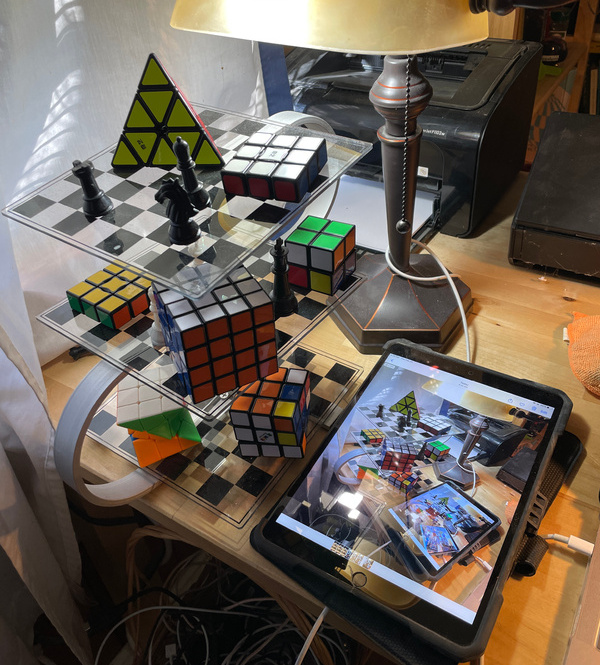Click for a large picture
Here is a short animation explaining the 2x2x1 puzzle
Here are two nice solutions from the class presenting the solution of the 3-puzzle (the 2x2 analogue of the 15 puzzle)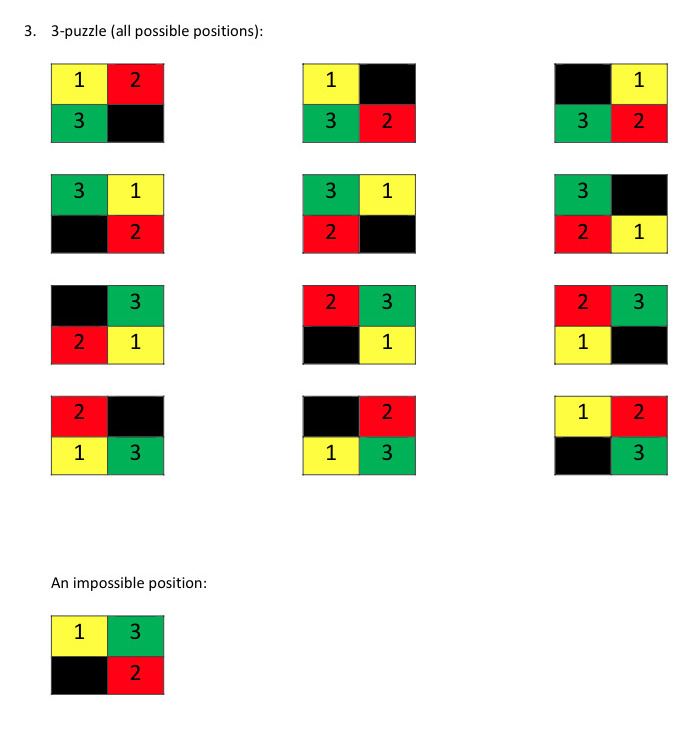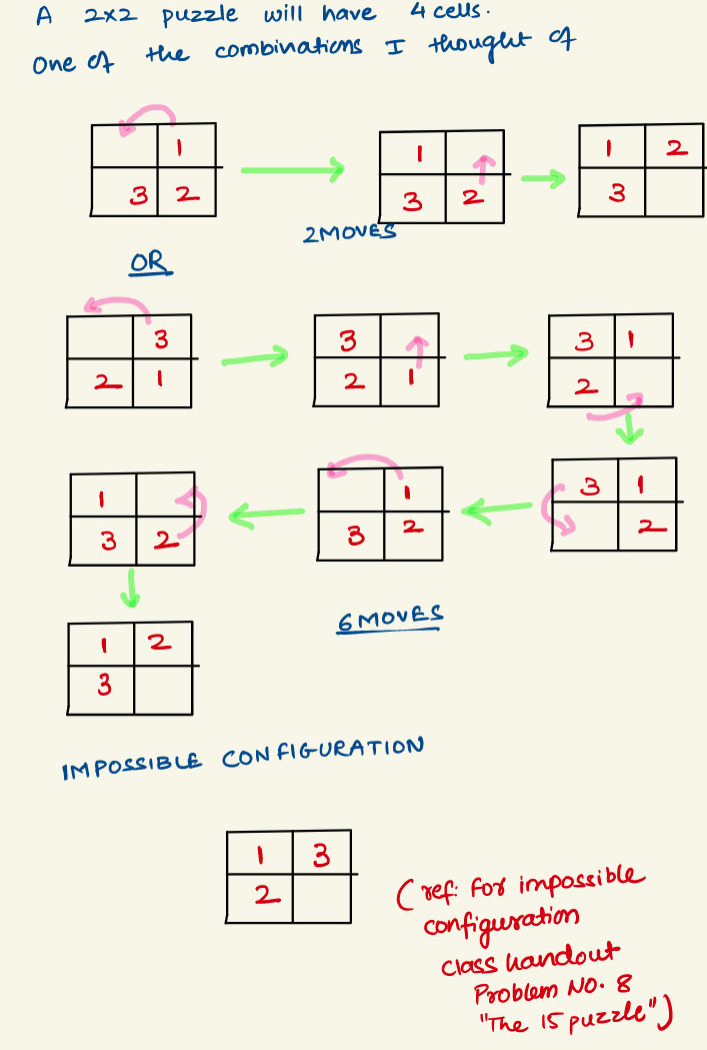#### Lecture 4: Number Theory

Update March 13, 2021: A new solution to x3+y3+z3=3.: 569936821221962380720^3 + (-569936821113563493509)^3 + (-472715493453327032)^3 = 3. Of course, there is the trivial solution x=y=z=1 and x=y=4, y=-5 because 64+64-125=2. Mordell had asked in 1953, whether there is an other one. This has now been found by Brooker and Sutherland: (article).
Top 10 in Number Theory
 Ranking Conjecture 1 Perfect 2 Landau 3 Goldbach 4 Twin 5 Riemann 6 ABC 7 Beal 8 Legendre 9 Andrica 10 Gilbreath
 Ranking Theorem 1 Fundamental 2 Fermat 3 Wilson 4 Prime number 5 Reciprocity 6 Christmas 7 Perfect 8 Lagrange 9 Dirichlet 10 Chinese
In the video, I briefly mentioned why the Riemann hypothesis RH could be wrong. Littlewood, who got the problem as a thesis problem (!) is rumored to have believed that it is wrong (maybe to protect himself from the embarresment not to be able to solve it ....). As a way to think intuitively about RH, already Littlewood has pointed to the fact if the Moebius function were ``random", the law of iterated log would give RH. Andrew Odlyzko (whom I met about 20 years ago when he visited the department and who has disproved the Mertens conjectures and has computed millions of zeros of zeta especially to get evidence for the Montgomery pair correlation conjecture), told me then about curious correlations for the Moebius function. The number theoretical functions which need to be ``random" might not be as random as they might need to be. The Hilbert-Polya conjecture (find a self-adjoint operator for which the spectrum give the roots) is also often given as evidence for RH. It has been tried by heavyweights like Connes. Odlyzko has some Historical pointers to the Hilbert-Polya point. See also this book written by experts on experimental math.
 P.S. Update February 23: The Moebius function was just mentioned in a new blog entry of Tao. I myself am very fond of the Moebius function. One reason is that it is a Poincare-Hopf index of a Morse build-up of the integers. (See my paper On Primes, Graphs and Cohomology. (I learned later that of Bjoerners work: A cell complex in number theory.) To the right is the geometric illustration of the number 30. All numbers until 30 are drawn. Two are connected if one is divisible by the other. When adding a new number, the Euler characteristic changes according to the value of the Moebius function. Understanding the growth rate of the Euler characteristic would settle RH. This picture combines number theory, combinatorial topology and differential topology (Morse theory). If the Moebius function were ``random", then the Riemann hypothesis would hold. It appears however that the Moebius function is not so random after all. Whether it is enough random for the law of iterated log is just the Riemann hypothesis. I personally tend to believe that RH is false also because we have seen many, many times, that wishful thinking in symmetries were wrong, parity (= reflection symmetry in space) in physics is an example, the latest is super symmetry, where the world best people have been completely wrong (The Large Hadron collider has not found super symmetry despite promises from the elite of physicists that it would be seen). If there is no compelling reason for a symmetry, it might well be broken.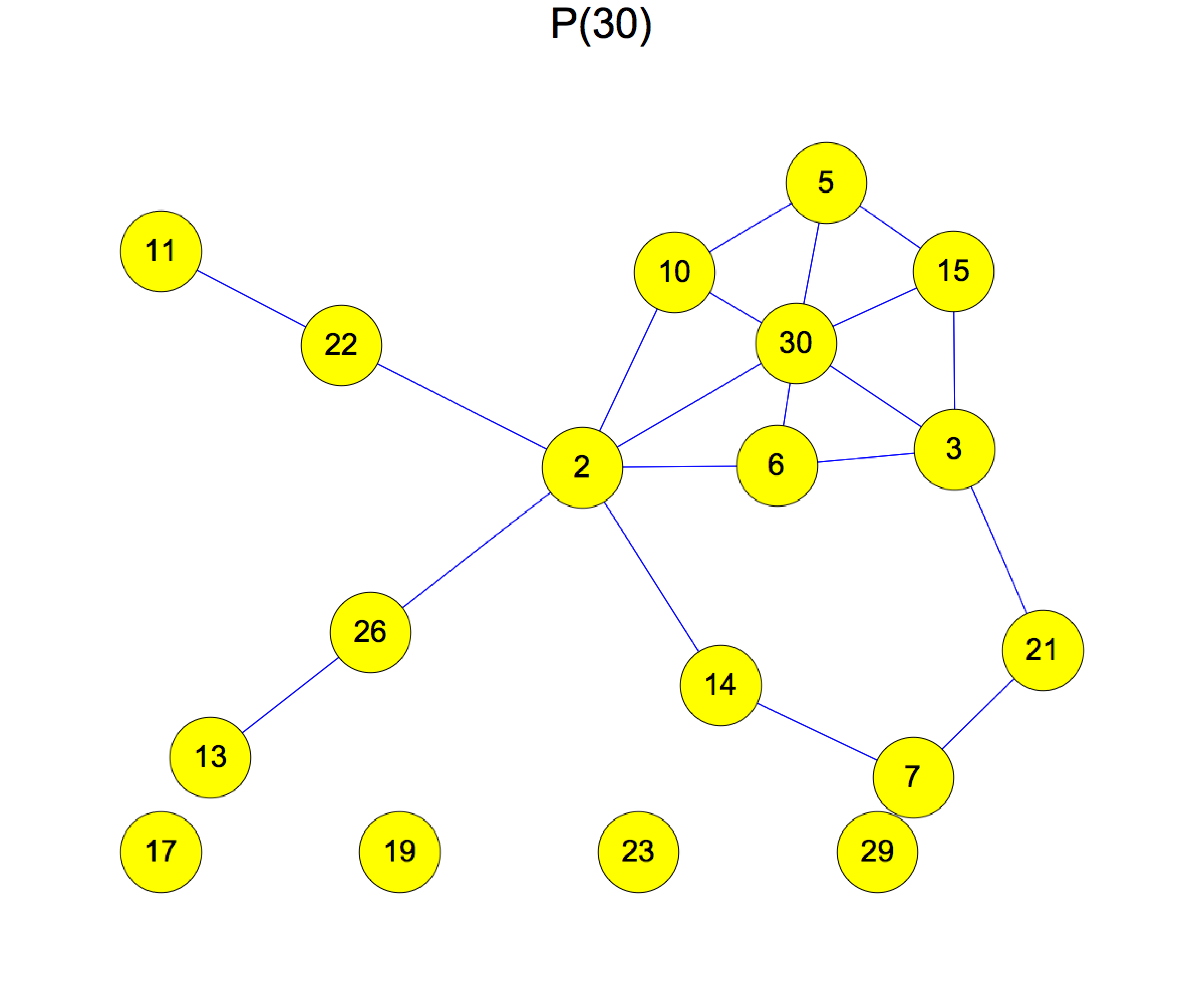Here is an illustration of the Gilbreath conjecture. The pictures look a bit like cellular automata. Indeed they are.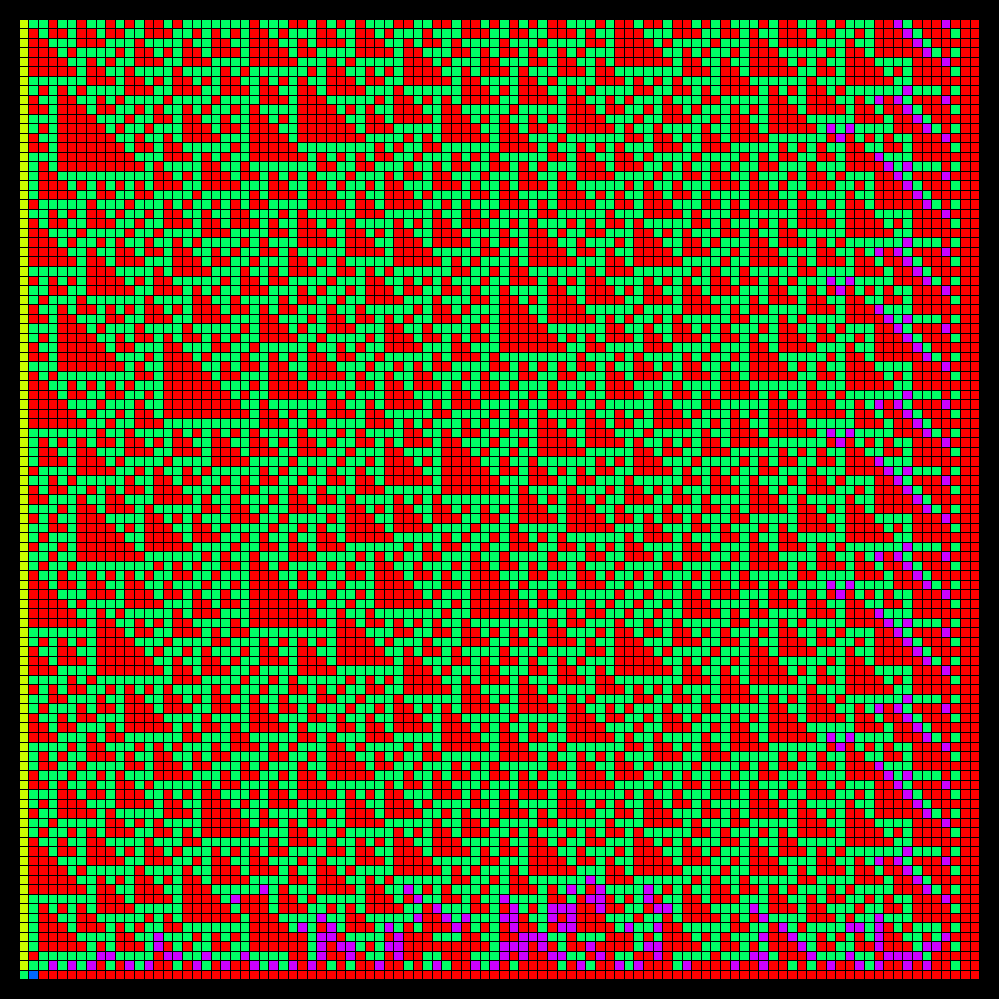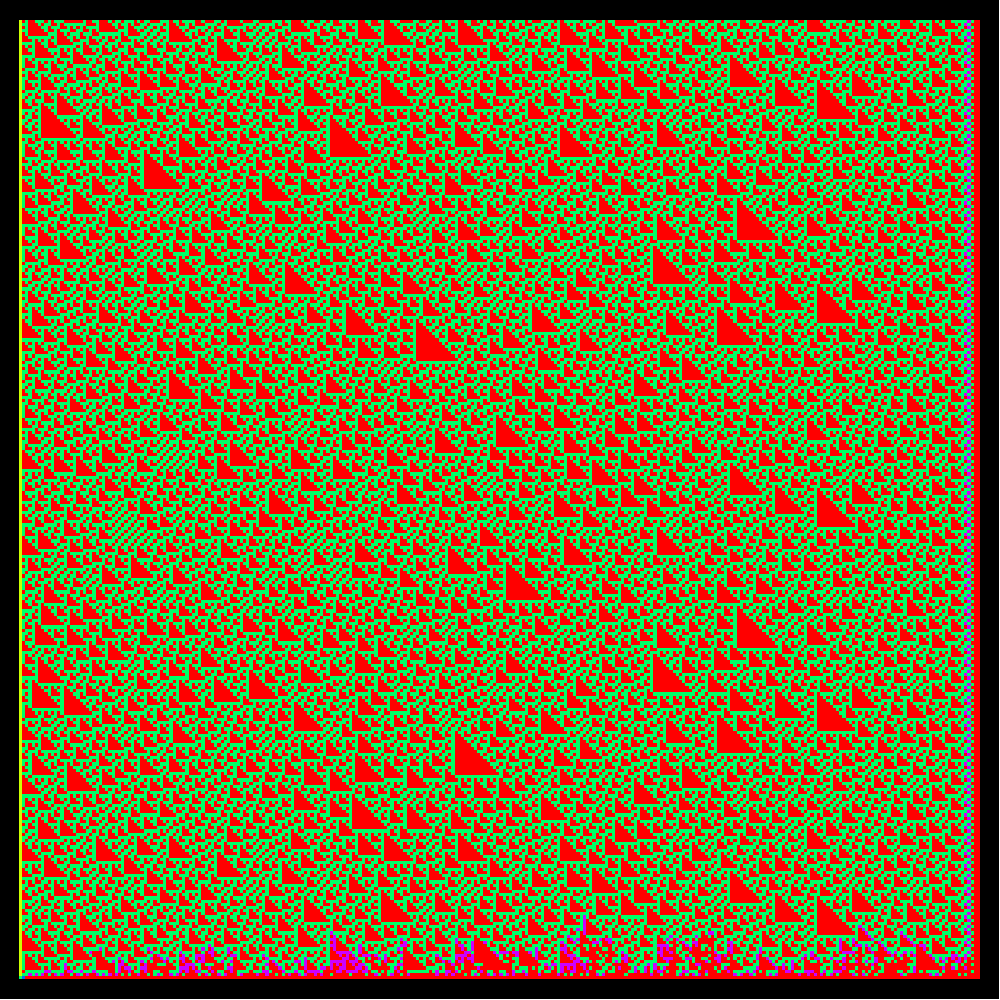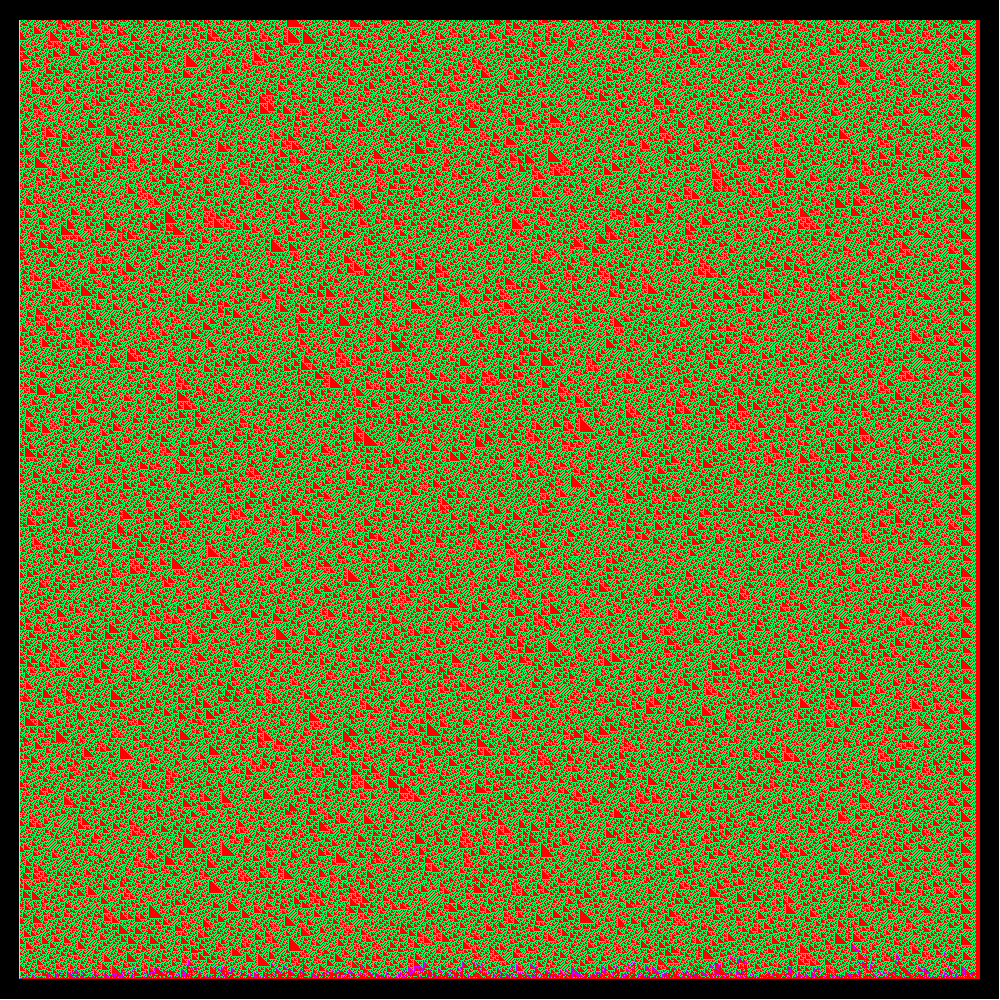In class, we mostly focused on primes, the Wilson and little Fermat theorem. The existence of arbitrary large prime gaps and the proof of Wilson's theorem were done in group. Very well. We had a bit little time left for the proof of the little Fermat theorem. Two parts of the presentation were done, one on open problems (which is covered in the pre-presentation below) and some unit on the Chinese remainder theorem, a topic which one can cover even pre-highschool level (I presented once in the Harvard math circle for kids). Here is a website about this from 2014. An exercise in the Mazur-Stein book prompted me to do some experiments in number theory.

#### Lecture 3: Geometry

• In the geometry lecture we have limited us mostly some theorems in good old planimetry. This had been done already 10 years ago, when starting this course and it is a rich enough play field. It is the first time that in group work Thales theorem was proven in teams, this year without much preparation. And many succeeded to prove it.
• Trying to defining geometry as a theory of shape, size and symmetry can be a bit of a stretch. It tries to incorporate that it deals with quantitative measurements (like area, length or volume) of objects in some space and then use Klein's Erlanger program as a guide to distinguish different geometries (like Euclidean geometry or projective geometry). In modern times, geometers often either algebraic geometers (often actually quite abstract commutative algebra or category theorists) or differential geometers (operating with rather heavy analytic machinery including partial differential equations). Closely related to geometry is topology (which we will cover later) and combinatorics, where the geometric objects are discrete. And then there is a growing area of computational geometry, especially related to computer science like in computer vision or game development. The geometric problems there are very concrete and often engineering type in the sense that one does not necessary aim for the most elegant solutions or beautiful theorems but for the fastest way to realize, simulate and navigate a given virtual world. The last century also has developed more and more non-commutative geometries. The passage is most natural when translating geometric problems first into algebra (like a commutative ring, as Descartes already did) and then see how much of the theory goes over to non-commutative rings like operator algebras. The theory of C* algebras for example is a natural generalization of topology and the theory of von Neumann algebras a natural generalization of probability theory. For geometry it is a bit more tricky and Connes extends it using spectral triples.
• There is a recent youtube video about Lockhart's famous article ``A Mathematician's Lament". In that video the Thales theorem is mentioned but again only in the case when the base is the diagonal. Here is again the animation of the Thales theorem. The animation had been written in 2010. I just upgraded it to 3D graphics:

Direct Media Links: Webm, Ogg Ipod

#### Lecture 2: Number Systems

Here is an other attempt to outline the structure of modern number systems. Some of the things are a bit strange at first, like the p-adic world. The interest there is mostly from number theory and algebra. We will talk in class about the metric completion of the rational numbers to the real numbers. That is something very natural as we see numbers like pi or the square root of 2 which are not rational numbers. One can however complete the rational numbers with respect to other distances and it turns out that there are not many such completions. There is one for every prime p and then there is the Archimedian completion which is given by the usual distance between numbers. We might quickly look at surreal numbers, introduced by John Conway which are also quite natural as they generalize to games. The hyper reals are used in non-standard mathematics. The quaternions and Octonions are natural as they complete the world of division algebras. Algebraic numbers are natural as these are numbers we can access with a finite amount of information, the integer coefficients of a polynomial. The modular arithmetic Z/(n) will appear for us later when we look at cryptology.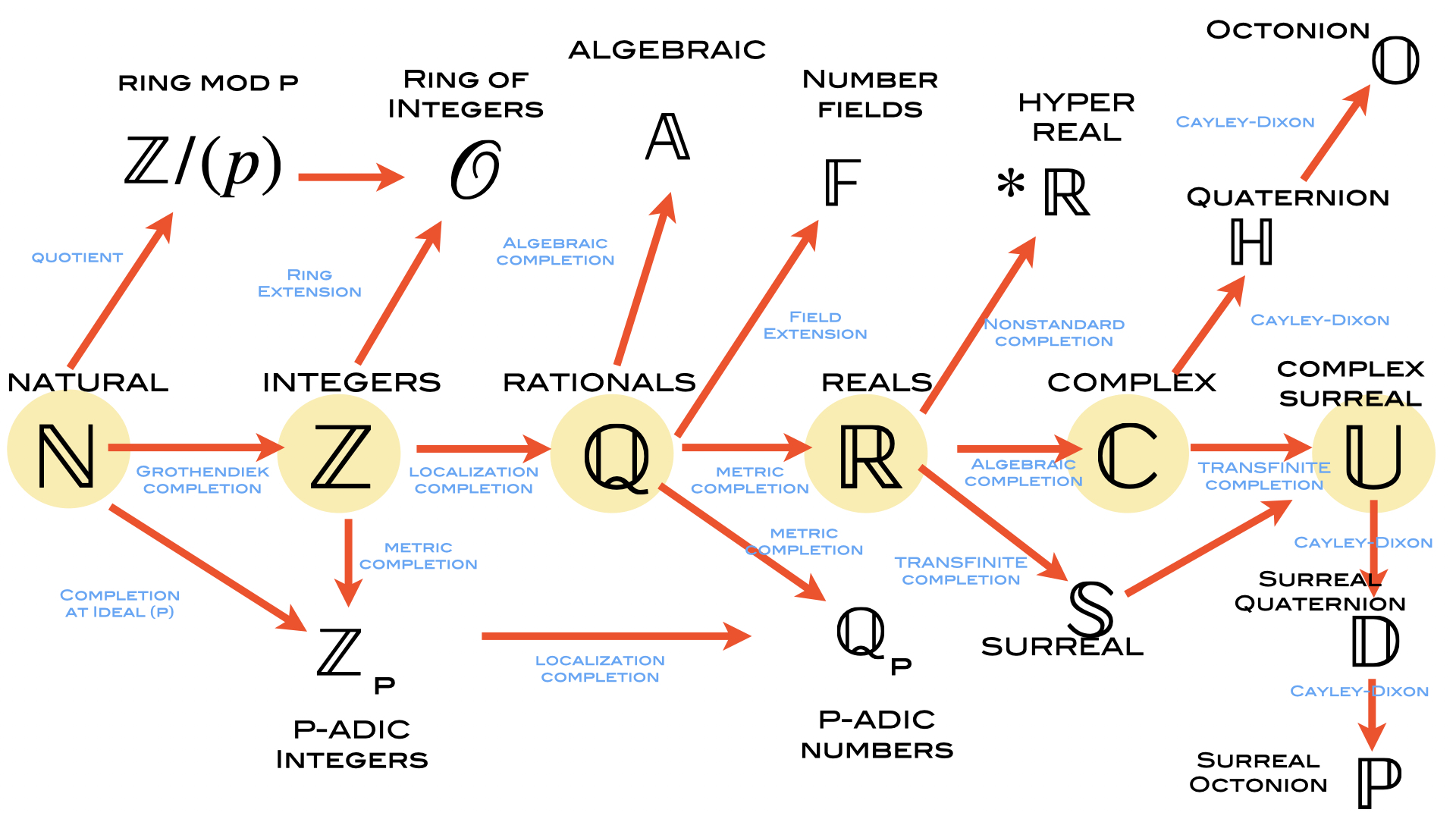As usual, the Wikipedia source on numbers is good with a picture illustrating the main number systems N,Z,Q,R,C. Finally, lets mention an extension of numbers to ``games". It is covered in a book of John Conway: ``Numbers and Games". Just to wet the appetite, here is a page from that book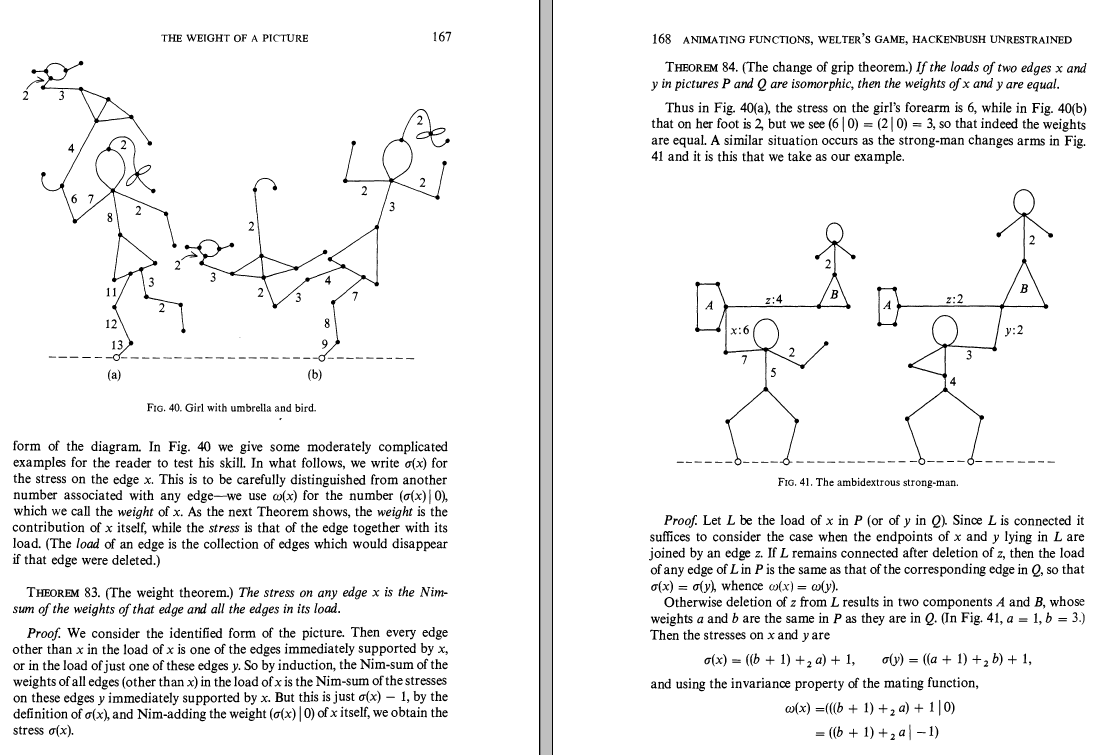#### Lecture 1: What is mathematics

 The world of map mentioned in class is here (youtube). We will actually cover almost all topics mentioned. Our own quick mindmap done today in class is to the right. About the taxonomy of the 7 liberal arts and sciences: there is an exhibit on the drawing of Harrad of Landsberg.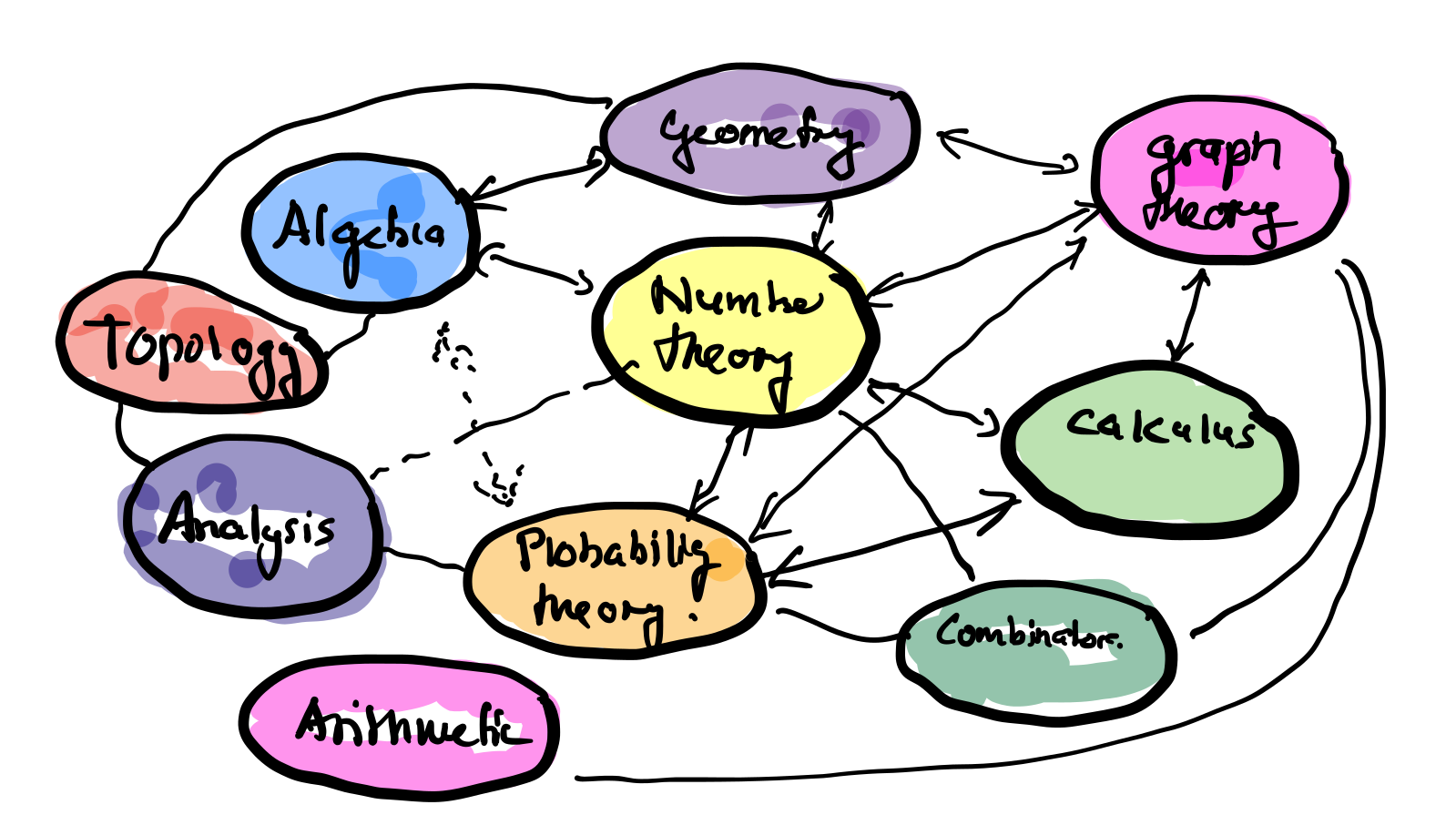Drawing the diagonals of a polygon seems to be a pretty dull thing to do. But it is very interesting. I myself worked over the winter break on this youtube slides. We looked at the triangle on page 8 of this document or simply click on the thumbnail to the right to see that part. Here is the worksheet.